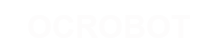#### Site Tools

en:reference:language:bitwisexornot

# Differences

This shows you the differences between two versions of the page.

 — en:reference:language:bitwisexornot [2017/04/07 10:03] (current) 2014/11/13 15:03 弘毅 created 2014/11/13 15:03 弘毅 created Line 1: Line 1: + ====== Bitwise NOT (~) ====== + + + The bitwise NOT operator in C++ is the tilde character ~. Unlike & and |, the bitwise NOT operator is applied to a single operand to its right. Bitwise NOT changes each bit to its opposite: 0 becomes 1, and 1 becomes 0. For example: + + 0  1    operand1 + ​---------- + 1  0   ~ operand1 + int a = 103;    // binary: ​ 0000000001100111 + int b = ~a;     // binary: ​ 1111111110011000 = -104 + ​ + You might be surprised to see a negative number like -104 as the result of this operation. This is because the highest bit in an int variable is the so-called sign bit. If the highest bit is 1, the number is interpreted as negative. This encoding of positive and negative numbers is referred to as two's complement. For more information,​ see the Wikipedia article on two's complement. + + + As an aside, it is interesting to note that for any integer x, ~x is the same as -x-1. + + + At times, the sign bit in a signed integer expression can cause some unwanted surprises. +